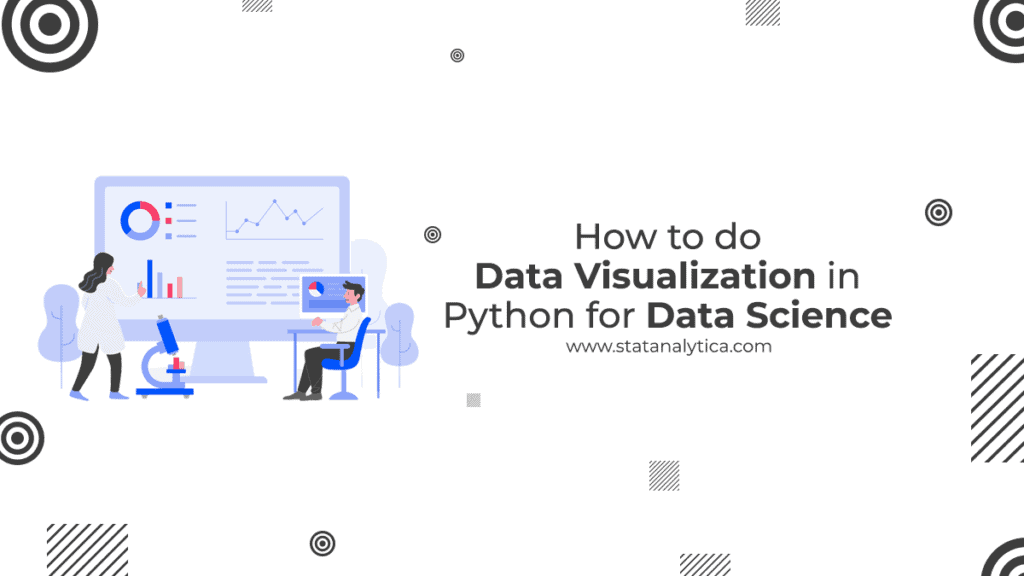# How to do Data Visualization in Python for Data Science

The graphical representation of data and information using various elements such as charts, graphs, maps, and other data visualization tools is called Data visualization. With the help of Data Visualization tools, it becomes easy to understand trends, patterns in data. It plays an important role in analyzing data or data science.

Python offers multiple graphing libraries that have multiple features. With the help of python libraries, it’s easy to perform data visualization. No matter what type of interactive plots you want to create, Python has a great library for you. So it is easy to Data Visualization in Python.

Data Science in Python is just data exploring and analyzing the python libraries and then turning data into colorful. Data Visualization includes Mataplotlib, Seaborn, Datasets, etc. machine learning is also a part of Data visualization defined as supervised and unsupervised learning tasks. Machine learning includes Scikit-learn, statsmodels. But this one is a deep learning subject and commonly implemented with Keras, TensorFlow, and a whole host of others.

In this blog, we will discuss How to do data visualization with Python for data science. With the help of python popular plotting libraries, we will perform data visualization.

## Data Visualization in Python

### Importing Datasets

With the help of two datasets, we will adapt the data visualizations freely available to access. In the Iris and Wine Reviews dataset, we can load them by using pandas. Two files are there temporal.csv and maps.csv. First, we will use the vast majority of tutorials with the popularity of data. And the next one mapa.csv, includes the popularity separated by country.

### Matplotlib

This one is the most popular Python plotting library at a low level, but it offers freedom at the cost of having to write more content. Mataplotib is most popular for creating graphs, line charts, bar diagrams, histograms etc. For installing pip and conda codes is used.

### Scatter Plot

For creating scatter plots under matplotlib, we have to use the scatter method. With the help of plt.subplots we can create a figure and plot title. With the help of highlighting data points, we can increase the meaning of graphs.

### Chart

charts play an important role in data visualization in Python. With plot methods, we can perform charts such as line charts and others in Matplotlib. You can plot two or more columns in one graph with looping through the columns.

### Histogram

The Hist method which performs the function of the histogram in Matplotlib. When we pass categorical data like the point column from the wine review dataset, it will automatically calculate how much every class occurs.

### Pandas Visualization

Pandas is a basic method of data visualization. It is used to get an idea of how to distribute. We have to visualize a few examples to check what columns there are, what type of information there, and how the different values are coded. The thing that bothers most while visualizing the data is the limited number of rows and columns in pandas. We will get more information when we view the tables. Besides, we can highlight the maximum and minimum value of the color on the table. We can also combine the functions and generate a more complex visualization.

### Pandas profiling

The library that generates reports with data can check the type of data distribution, possible problems, that is profiling data. It is so easy to generate a report in three lines and send it to anyone that can be used even if we don’t know about programming.

Similarly, Matplotlib, we have to create a scatter plot, line chart, Bar chart, histogram in Pandas for the Data visualization in Python.

### Seaborn library

The Seaborn library is also an indicator of data visualization with Python for data science. For designing attractive graphs of high level seaborn is required. In comparison to Matplotlib, the Seaborn is more useful because while you are preparing charts, Seaborn takes less time than the Matplotib and work attractively. You can create graphs in one line.

It can be imported with Import Seaborn as sns. As Pandas, we need to use scatter methods for creating Scatter Plot, but now you don’t need to pass data from columns, because we already do in Pandas.

To create a chart, you should perform sns.lineplot method; for Bar chart, you should go through sns.countplot method and pass out the data.

## Some Basic Data Visualization Tools

We already studied the important data visualization tools above. Here are some basic visualization tools that help you extract from insides and visualize the data with Python.

### Faceting

It breaks the data and then combines them in a single figure. Faceting is more useful when we use Facegrid in Seaborn.

### Heatmap

The graphical representation of data where values are mentioned is represented in colors. Perfect for exploring datasets, also used in matplotlib and seaborn.

### The box plots

It is also a graphical representation of data for data visualization in Python for data science. Box plots are created with the help of seaborn. They are just similar to the bar charts.

It is all about how you can utilize Data Visualization in Python for Data Science.

## Conclusion

From this article, we know that how much Data Visualisation in Python is important for data science. Python offers multiple graphing libraries with different advanced features. We discuss all the important terms such as Matplotlib, pandas visualization, seaborn and many other basic or specific tools. If you need any help related to the assignment of python programming then take the python assignment help and Python assignment service from our experts to